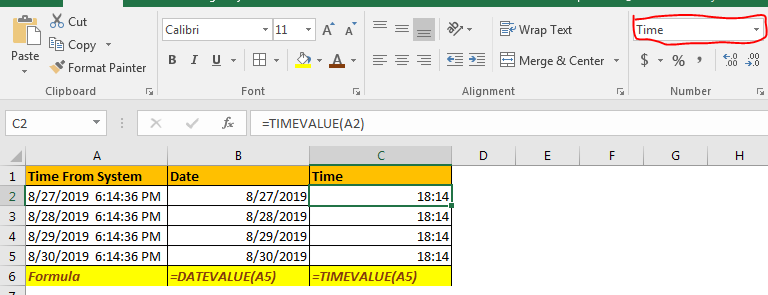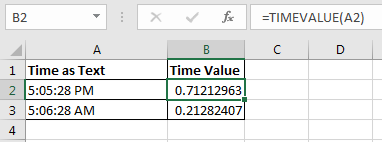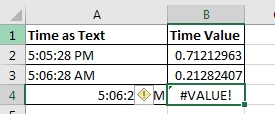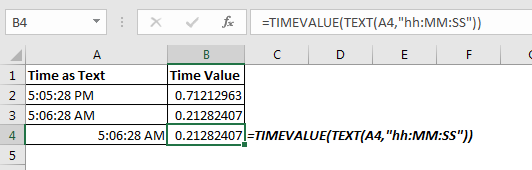# How To Use TIMEVALUE Function in ExcelThe TIMEVALUE simply converts the text time into decimal equivalent of the time. The input should be a time that is formatted as text.
Syntax:

=TIMEVALUE(time_text)

Time_text: The time_text is a time that is formatted as text. For example “15:52” or “4:45:00 PM” or “4:45 PM” or “8/27/2019 4:53:43 PM”. All these are valid inputs for TIMEVALUE function. Any other text or value will generate #VALUE error.
How to use TIMEVALUE function
Supply time_text hard coded to TIMEVALUE
You can supply hardcode time to get its decimal equivalent. It just needs to be enclosed in double quotes.

=TIMEVALUE(“3:00 PM”)

Above TIMEVALUE formula will return 0.625.
Supply as Cell Reference
If you have time as text in a cell, you can forward that cell as reference to TIMEVALUE function of Excel.

=TIMEVALUE(A1)Make sure that, time in given cell is a text. If the time in given cell is not a text, it will return a #VALUE error.If you want still want to get time value from time that is not formated as text, use below TIMEVALUE formula.

=TIMEVALUE(TEXT(A4,"HH:MM:SS"))Here, we used TEXT function to convert time into text and then we use TIMEVALUE function to get its time value.
TIMEVALUE Example: Separate Time and Date
Most of the the software generated data is text formatted. When you receive data that is not have data of its appropriate type, it gets hard to crunch that data and make meaningful reports. Specially times.
Assume that a system give us below time data.Now, we need to split time and date in different columns. You can of course Extract Text From A String In Excel Using Excel’s LEFT And RIGHT Function but that’s little bit tricky and it will return a text value. Using DATEVALUE and TIME VALUE it can be don easily. Let’s see how.

If your data is already a text formatted then, you just need to write this TIMEVALUE Function to get time in C2 cell.

=TIMEVALUE(A2)

Now format that cell as time and you will have your time in C column. The formula ignores the date part.

Similarly in B1 write this DATEVALUE formula.

=DATEVALUE(A2)

This will return a number that represents a date. Change the cell format as Date. This formula ignores the time part.

So yeah friends, this is the TIMEVALUE function of excel. I hope this was helpful. If you have any query, let me know in the comments section below. Till then here are some useful related articles.

Related Articles:

Separating Date and Time

Convert Date and Time from GMT to CST

Calculate Minutes Between Dates & Time In Microsoft Excel

Calculate hours between time in Excel

Multiplying time values and numbers.

Excel convert decimal Seconds into time format

Convert Time to Decimal in Excel

Text to Time Conversion

Popular Articles:

50 Excel Shortcuts to Increase Your Productivity

How to use the VLOOKUP Function in Excel

How to use the COUNTIF in Excel 2016

How to use the SUMIF Function in Excel

Terms and Conditions of use

The applications/code on this site are distributed as is and without warranties or liability. In no event shall the owner of the copyrights, or the authors of the applications/code be liable for any loss of profit, any problems or any damage resulting from the use or evaluation of the applications/code.